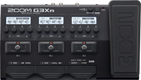# Banbeg

Discussion in 'Zoom G3n/G3Xn/G5n' started by Banbeg, Dec 31, 2022.

1. BanbegDevice: Zoom G3Xn
Firmware: 2.20

Name on device: Day Trippy
Optimized for: Guitar Amp

Effects chain:beatles

Effect: "ZNR" (Dynamics), active - "yes"
"Detect" = EFXIN
"Depth" = 100
"Threshold" = 45
"Decay" = 0

Effect: "Comp" (Dynamics), active - "yes"
"Sense" = 6
"Attack" = Slow
"Tone" = 5
"Volume" = 91

Effect: "Gt GEQ 7" (Filter), active - "yes"
"100 Hz" = 0.5
"200 Hz" = 0.5
"400 Hz" = 1.0
"800 Hz" = 0.5
"1.6 kHz" = 0.0
"3.2 kHz" = 1.5
"6.4 kHz" = -1.0
"Volume" = 81
"100 Hz" = 0.5
"200 Hz" = 1.5
"400 Hz" = -1.0
"800 Hz" = -1.0
"1.6 kHz" = 0.0
"3.2 kHz" = -0.5
"6.4 kHz" = 0.0
"Volume" = 80
"Parameter set" = B

Effect: "UK 30A" (Amp simulator), active - "yes"
"Bass" = 31
"Treble" = 36
"Cut" = 72
"Gain" = 17
"Volume" = 83
"Depth" = 0
"Speed" = 50
"SOLO" = 1

Effect: "Plate" (Reverb), active - "yes"
"PreD" = 2
"Decay" = 7
"Mix" = 44
"Tail" = Off

Patch Volume: 100